Categories

## Sets and Integers | TOMATO B.Stat Objective 121

Try this problem from I.S.I. B.Stat Entrance Objective Problem based on Sets and Integers.

## Sets and Integers ( B.Stat Objective Question )

For each positive integer n consider the set $S_n$ defined as follows $S_1$={1}, $S_2$={2,3}, $S_3$={4,5,6}, …, and , in general, $S_{n+1}$ consists of n+1 consecutive integers the smallest of which is one more than the largest integer in $S_{n}$. Then the sum of all the integers in $S_{21}$ equals

• 1113
• 4641
• 53361
• 5082

### Key Concepts

Sets

Integers

Sum

B.Stat Objective Problem 121

Challenges and Thrills of Pre-College Mathematics by University Press

## Try with Hints

First hint

$S_1$ has 1 element

$S_2$ has 2 element

…..

$S_{20}$ has 20 element

Second Hint

So number of numbers covered=1+2+3+…+20

sum =$\frac{(20)(21)}{2}$=210

$S_{21}$ has 21 elements with first element= 211

Final Step

sum of n terms of a.p series with common difference d,

$sum=\frac{n}{2}[2a+(n-1)d]$

Then in our given question no of terms n=21 and c.d =1

Then the sum of elements=$\frac{21}{2}[(2)(211)+(20)(1)]$=4641.

Categories

## Problem on Digits | TOMATO B.Stat Objective 111

Try this problem from I.S.I. B.Stat Entrance Objective Problem based on Problem on Digits.

## Problem on Digits ( B.Stat Objective Question )

The sum of all the distinct four digit numbers that can be formed using the digits 1, 2, 3, 4, 5 each digit appearing at most once is

• 399900
• 399960
• 0
• none of these

### Key Concepts

Digits

Sum

Non-repeated

B.Stat Objective Problem 111

Challenges and Thrills of Pre-College Mathematics by University Press

## Try with Hints

First hint

here every strings are 1234, 2345, 1345, 1235, 2345

Second Hint

for 1234, sum =66660

for 1235, sum=66+660+6600+66000=73326

for 2345, sum=84+840+8400+84000=93324

for 1345, sum=78+780+7800+78000=86658

for 1245, sum=72+720+7200+72000=79992

Final Step

then 66660+93324+73326+79992+86658=399960.

Categories

[h5p id="14"]

# Understand the problem

Let$a_n = n+\frac{1}{n} , n \in \mathbb{N}$. Then the sum of the series$\sum_{n=1}^{\infty} (-1)^{n+1} \frac{a_{n+1}}{n!} is$ (A)$e^{-1}-1$ (B)$e^{-1}$ (C)$1-e^{-1}$ (D)$1+e^{-1}$
##### Source of the problem
IIT JAM 2018 Problem 13
Series
Easy
##### Suggested Book
Real Analysis By S.K Mapa

Do you really need a hint? Try it first!

Consider$a_n = n + \frac{1}{n} , n \in \mathbb{N}$ We have to use$e^{x} = 1 + \frac{x}{1!} + \frac{x^{2}}{2!}+.....$ Specifically$e^{1} = 1+ \frac{1}{1!} + \frac{1}{2!}+....$ And$e^{-1} = 1 - \frac{1}{1!} + \frac{1}{2!} - \frac{1}{3!} + .......$ Do you want to play with it$\sum_{n=1}^{\infty} (-1)^{n+1} \frac{a_{n+1}}{n!} = \sum_{n=1}^{\infty} (-1)^{n+1} \frac{n+1 + \frac{1}{n+1}}{n!}$ =$\sum_{n=1}^{\infty}[ (-1)^{n+1} \frac{1}{(n-1)!} + (-1)^{n+1} \frac{1}{n!} + (-1)^{n+1} \frac{1}{(n+1)!}]$   Now we will be breaking it term by term for the ease of calculation. Can you do it from here?$\sum_{n=1}^{\infty} (-1)^{n+1} \frac{a_{n+1}}{n!}$ =$[1-\frac{1}{1!} + \frac{1}{2!} - ......] + [\frac{1}{1!} - \frac{1}{2!} + \frac{1}{3!} - .....] + [\frac{1}{2!} - \frac{1}{3!} + \frac{1}{4!} -.......]$ =$e^{-1} + [1-e^{-1}] + [e^{-1} + 1 - 1]$ =$e^{-1} + 1$ So option (D) is our required answer.

# The will look more easy if we take a look into the knowledge graph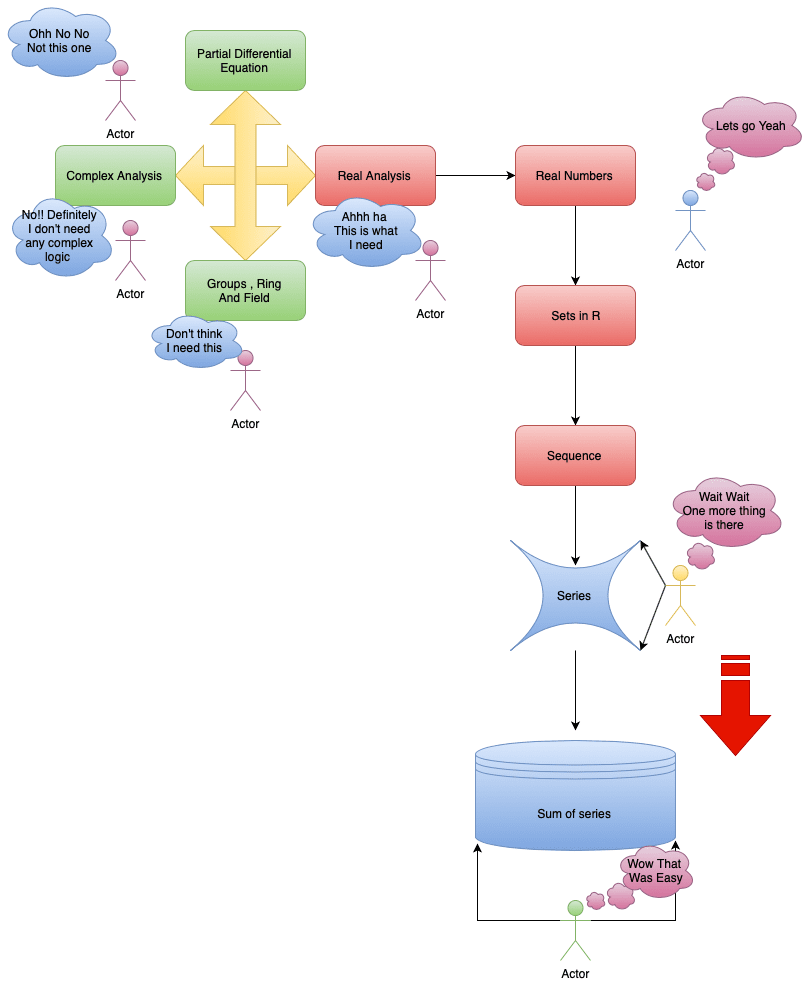# Let’s have a look into the graphs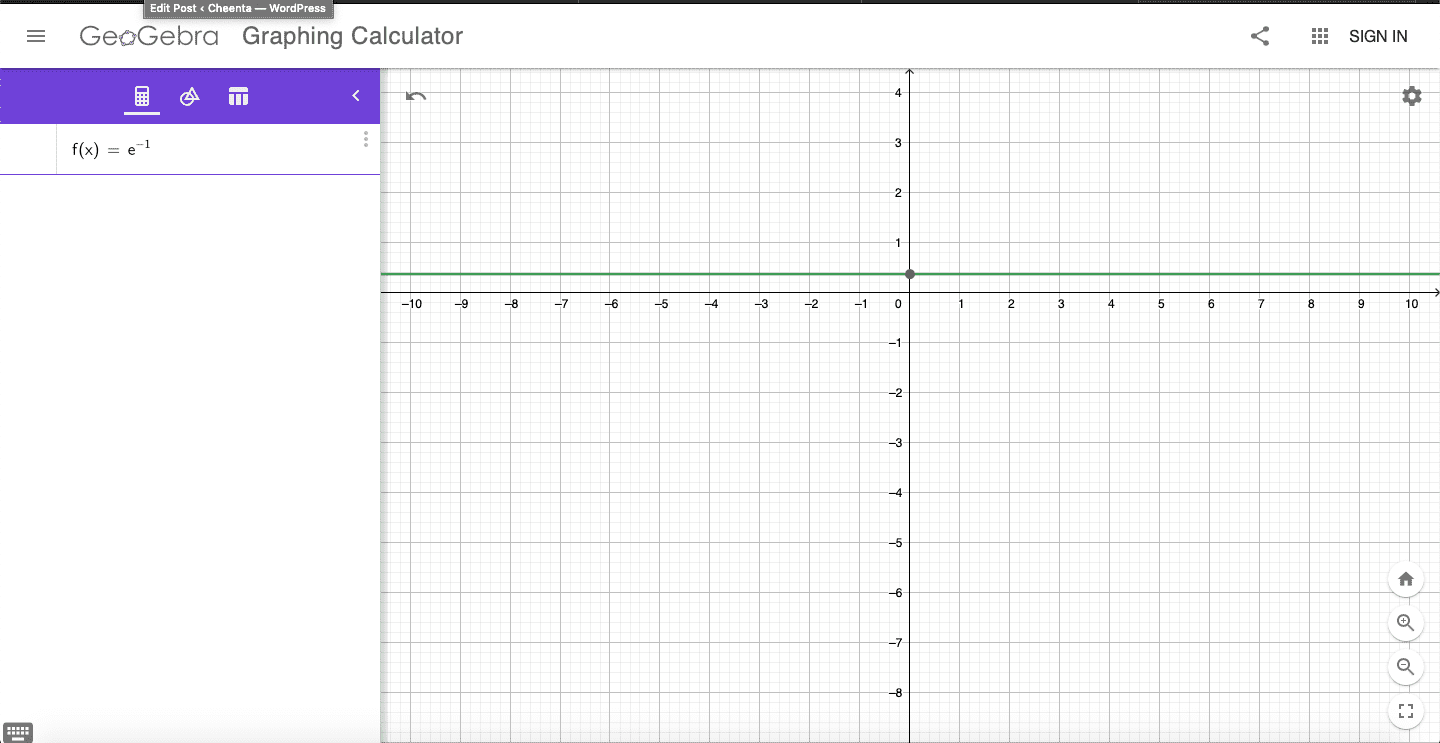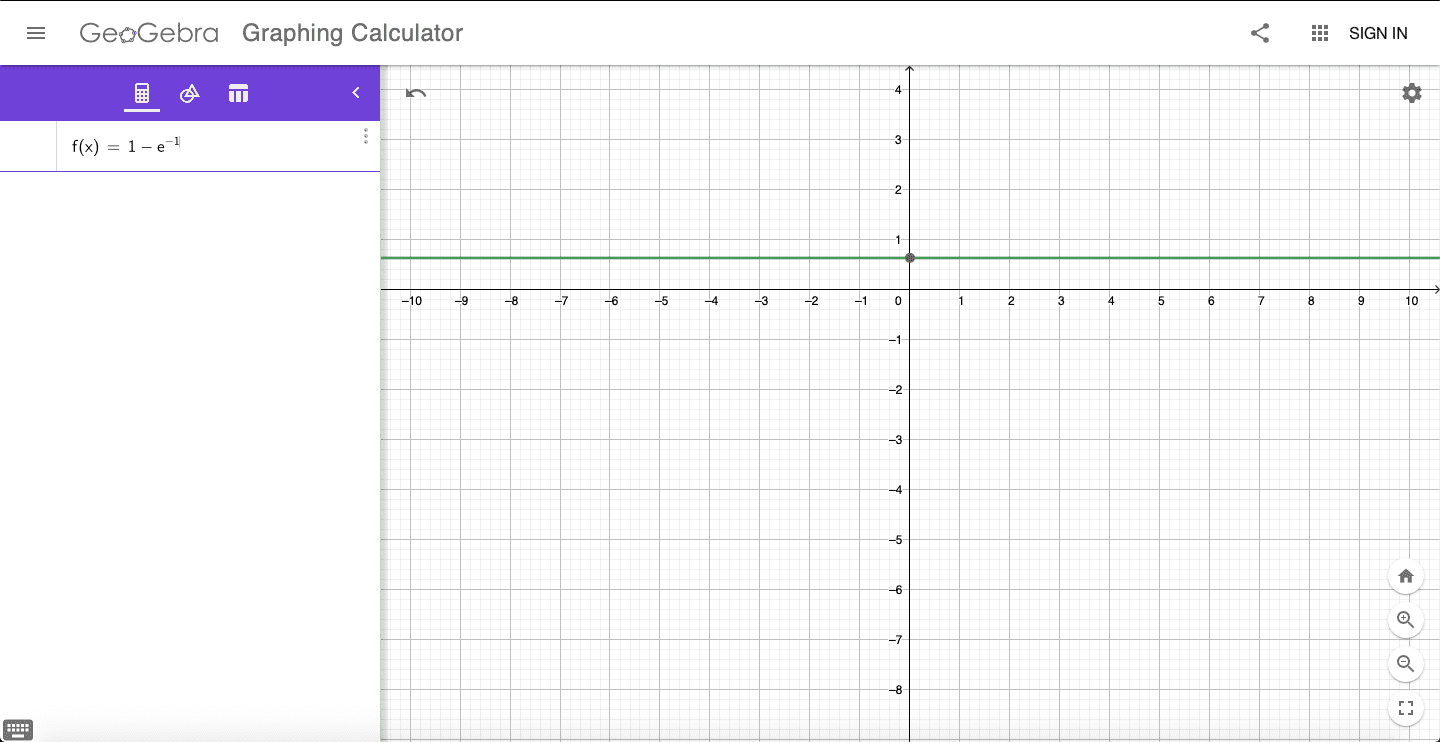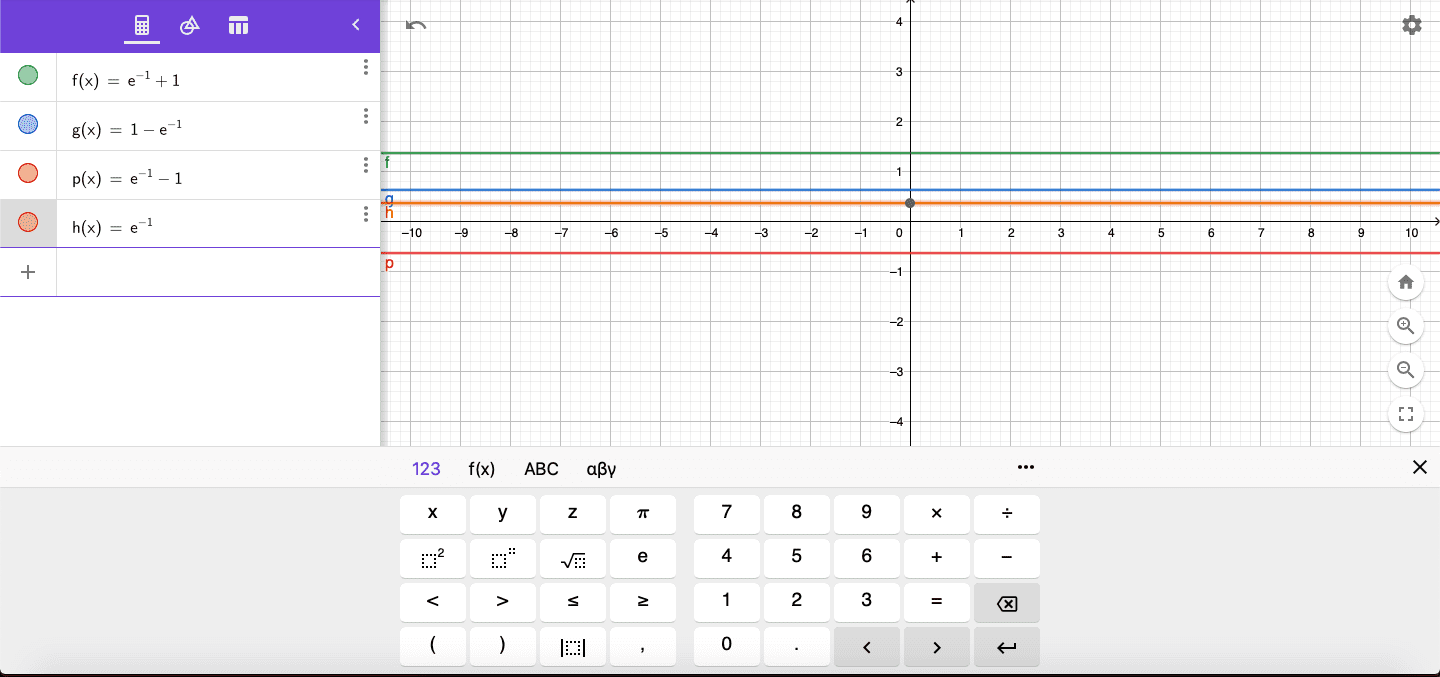# Do You Know ????# Connected Program at Cheenta

#### College Mathematics Program

The higher mathematics program caters to advanced college and university students. It is useful for I.S.I. M.Math Entrance, GRE Math Subject Test, TIFR Ph.D. Entrance, I.I.T. JAM. The program is problem driven. We work with candidates who have a deep love for mathematics. This program is also useful for adults continuing who wish to rediscover the world of mathematics.

# Similar Problems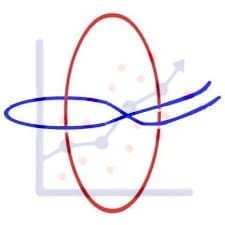## ISI MStat PSB 2006 Problem 8 | Bernoullian Beauty

This is a very simple and regular sample problem from ISI MStat PSB 2009 Problem 8. It It is based on testing the nature of the mean of Exponential distribution. Give it a Try it !## ISI MStat PSB 2009 Problem 8 | How big is the Mean?

This is a very simple and regular sample problem from ISI MStat PSB 2009 Problem 8. It It is based on testing the nature of the mean of Exponential distribution. Give it a Try it !## ISI MStat PSB 2009 Problem 4 | Polarized to Normal

This is a very beautiful sample problem from ISI MStat PSB 2009 Problem 4. It is based on the idea of Polar Transformations, but need a good deal of observation o realize that. Give it a Try it !## ISI MStat PSB 2009 Problem 6 | abNormal MLE of Normal

This is a very beautiful sample problem from ISI MStat PSB 2009 Problem 6. It is based on the idea of Restricted Maximum Likelihood Estimators, and Mean Squared Errors. Give it a Try it !## ISI MStat PSB 2009 Problem 3 | Gamma is not abNormal

This is a very simple but beautiful sample problem from ISI MStat PSB 2009 Problem 3. It is based on recognizing density function and then using CLT. Try it !## ISI MStat PSB 2009 Problem 1 | Nilpotent Matrices

This is a very simple sample problem from ISI MStat PSB 2009 Problem 1. It is based on basic properties of Nilpotent Matrices and Skew-symmetric Matrices. Try it !## ISI MStat PSB 2006 Problem 2 | Cauchy & Schwarz come to rescue

This is a very subtle sample problem from ISI MStat PSB 2006 Problem 2. After seeing this problem, one may think of using Lagrange Multipliers, but one can just find easier and beautiful way, if one is really keen to find one. Can you!

## Problem on Inequality | ISI – MSQMS – B, 2018 | Problem 2a

Try this problem from ISI MSQMS 2018 which involves the concept of Inequality. You can use the sequential hints provided to solve the problem.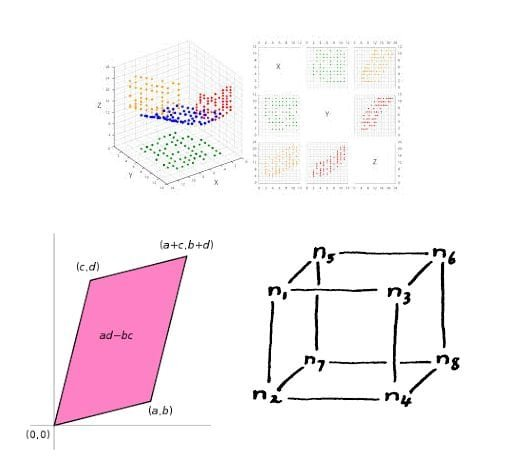## Data, Determinant and Simplex

This problem is a beautiful problem connecting linear algebra, geometry and data. Go ahead and dwelve into the glorious connection.

## Problem on Integral Inequality | ISI – MSQMS – B, 2015

Try this problem from ISI MSQMS 2015 which involves the concept of Integral Inequality and real analysis. You can use the sequential hints provided to solve the problem.